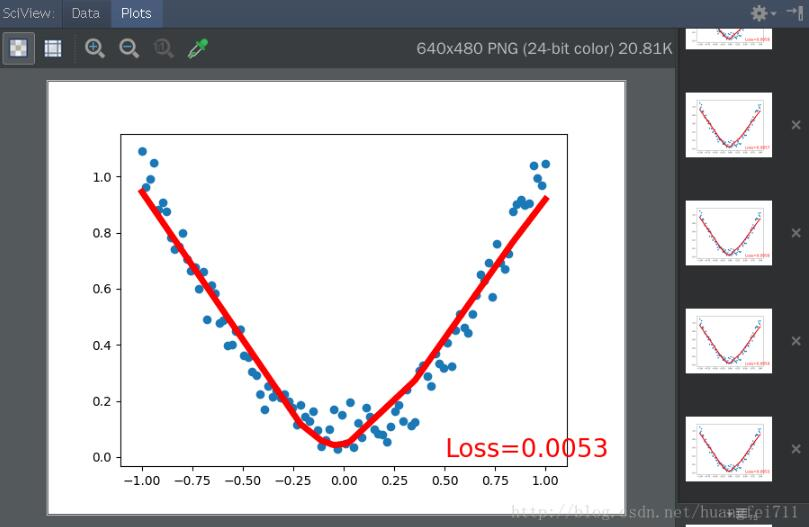# pytorch 模拟关系拟合――回归实例

更新时间：2020年01月14日 14:56:30   作者：HF飞哥我要评论

1. 建立数据集

```import torch
import matplotlib.pyplot as plt

# torch.linspace(-1,1,100)表示返回一个一维张量，包含在区间 -1到1 上均匀间隔的100个点；
# torch.unsqueeze(input,dim=1)表示转换维度
x = torch.unsqueeze(torch.linspace(-1, 1, 100), dim=1)
# 生成的y值为x的平方加上随机数
y = x.pow(2) + 0.2*torch.rand(x.size())

# 用 Variable 来修饰这些数据 tensor

# 画图
plt.scatter(x.data.numpy(), y.data.numpy())
plt.show()
```

2. 构建神经网络

```import torch
import torch.nn.functional as F   # 激励函数都在这

class Net(torch.nn.Module): # 继承 torch 的 Module
def __init__(self, n_feature, n_hidden, n_output):
super(Net, self).__init__()   # 继承 __init__ 功能
# 定义每层用什么样的形式
self.hidden = torch.nn.Linear(n_feature, n_hidden)  # 隐藏层线性输出
self.predict = torch.nn.Linear(n_hidden, n_output)  # 输出层线性输出

def forward(self, x):  # 这同时也是 Module 中的 forward 功能
# 正向传播输入值, 神经网络分析出输出值
x = F.relu(self.hidden(x))   # 激励函数(隐藏层的线性值)
x = self.predict(x)       # 输出值
return x

net = Net(n_feature=1, n_hidden=10, n_output=1)

print(net) # net 的结构
"""
Net (
(hidden): Linear (1 -> 10)
(predict): Linear (10 -> 1)
)
"""
```

3. 实时绘图查看回归效果

```import matplotlib.pyplot as plt

plt.ion() #打开交互绘图模式（便于实时显示图像变化）
plt.show()

optimizer = torch.optim.SGD(net.parameters(), lr=0.1) # 定义优化器和学习率
loss_func = torch.nn.MSELoss() #定义损失函数

for t in range(200):
prediction = net(x)
loss = loss_func(prediction, y)

loss.backward()
optimizer.step()

if t%5 == 0:
plt.cla()
plt.scatter(x.data.numpy(), y.data.numpy()) # 画散点图
plt.plot(x.data.numpy(), prediction.data.numpy(), 'r-', lw=5) # 画拟合曲线
plt.text(0.5, 0, 'Loss=%.4f' % loss.data, fontdict={'size':20,'color':'red'}) # 显示损失数值
plt.pause(0.1)

# 如果在脚本中使用ion()命令开启了交互模式，没有使用ioff()关闭的话，则图像会一闪而过，并不会常留。要想防止这种情况，需要在plt.show()之前加上ioff()命令。
plt.ioff()
plt.show()
```